# C++20: Concepts – Syntactic Sugar

Today, my post is not about something new to concepts. It’s about syntactic sugar. I write about abbreviated function templates. What? Abbreviated function templates allow a sweet way to define templates.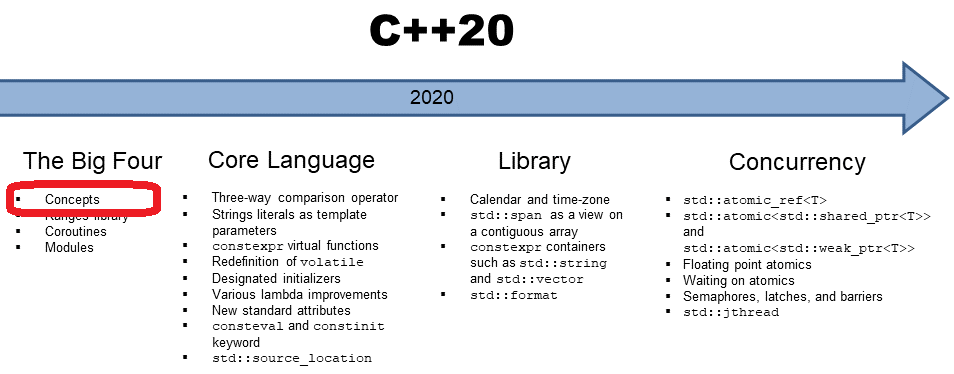First of all, here is the definition of syntactic sugar from Wikipedia:

In computer science, syntactic sugar is syntax within a programming language that is designed to make things easier to read or express. It makes the language “sweeter” for human use: things can be expressed more clearly, concisely, or in an alternative style that some may prefer.

The syntactic sugar I’m writing today is called abbreviated function templates.

##Modernes C++ Mentoring

Be part of my mentoring programs:

• "Fundamentals for C++ Professionals" (open)
• "Design Patterns and Architectural Patterns with C++" (open)
• "C++20: Get the Details" (reopens December 2023)
• Do you want to stay informed about my mentoring programs: Subscribe via E-Mail.

## Abbreviated Function Templates

I wrote in my last post about concepts C++20: Concepts, the Placeholder Syntax that we have had since C++14, a significant asymmetry: generic lambdas introduced a new way to define function templates. Just use auto as a function parameter. On the contrary, you can not use auto as a function parameter to get a function template.

```// genericLambdaFunction.cpp

#include <iostream>
#include <string>

auto addLambda = [](auto fir, auto sec){ return fir + sec; }; // (1)

auto addFunction(auto fir, auto sec){ return fir + sec; }     // (2)

int main(){

std::cout << std::boolalpha << std::endl;

std::cout << addLambda(1, 5) << " " << addFunction(1, 5) << std::endl;
std::cout << addLambda(true, 5) << " " << addFunction(true, 5) << std::endl;
std::cout << addLambda(1, 5.5) << " " << addFunction(1, 5.5) << std::endl;

const std::string fir{"ge"};
const std::string sec{"neric"};
std::cout << addLambda(fir, sec) << " " << addFunction(fir, sec) << std::endl;

std::cout << std::endl;

}
```

The Clang compiler speaks clear language when I try to compile this program with the C++14 standard.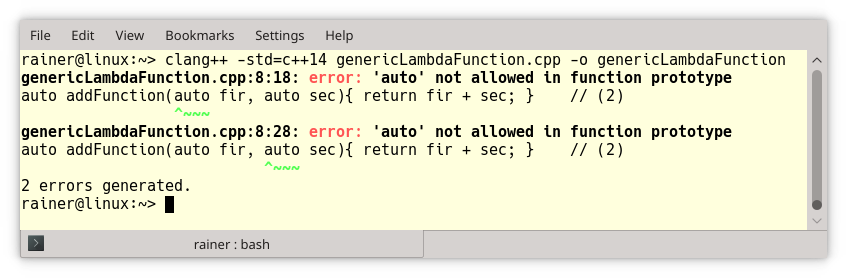How strange! I can use auto as the return type and for the function parameters in a lambda (line 1), but I can only use auto as the return type of a function (line 2).

I’m happy to say. This weird behavior is gone with C++20, and the unification is extended to concepts.

```// conceptsIntegralVariationsDraft.cpp

#include <type_traits>
#include <iostream>

template<typename T>                                  // (1)
concept Integral = std::is_integral<T>::value;

template<typename T>                                  // (2)
requires Integral<T>
T gcd(T a, T b){
if( b == 0 ) return a;
else return gcd(b, a % b);
}

template<typename T>                                  // (3)
T gcd1(T a, T b) requires Integral<T>{
if( b == 0 ){ return a; }
else return gcd(b, a % b);
}

template<Integral T>                                  // (4)
T gcd2(T a, T b){
if( b == 0 ){ return a; }
else return gcd(b, a % b);
}

Integral auto gcd3(Integral auto a, Integral auto b){ // (5)
if( b == 0 ){ return a; }
else return gcd(b, a % b);
}

auto gcd4(auto a, auto b){                            // (6)
if( b == 0 ){ return a; }
return gcd(b, a % b);
}

int main(){

std::cout << std::endl;

std::cout << "gcd(100, 10)= "  <<  gcd(100, 10)  << std::endl;
std::cout << "gcd1(100, 10)= " <<  gcd1(100, 10)  << std::endl;
std::cout << "gcd2(100, 10)= " <<  gcd2(100, 10)  << std::endl;
std::cout << "gcd3(100, 10)= " <<  gcd3(100, 10)  << std::endl;
std::cout << "gcd4(100, 10)= " <<  gcd3(100, 10)  << std::endl;

std::cout << std::endl;

}
```

Let me describe in a few words the already known stuff for my previous posts to concepts. Line 1 defines the concept Integral. gcd – gcd2 (lines 2 – 4) use the concept in various ways. gcd used the required clause, gcd1 the so-called trailing requires clause, and gcd2 constrained template parameters.

With gcd3, the syntactic sugar starts. The function declaration Integral auto gcd3(Integral auto a, Integral auto b) requires from its type parameters that they support the concept Integral. This syntactic form is the new way to use a concept and get a function template equivalent to the previous versions gcd – gcd2.

The syntactic form of gcd3 and gcd4 is called abbreviated function templates. Integral auto in the declaration of gcd3 is a  constrained placeholder (concept), but you can also use an unconstrained placeholder (auto)  in a function declaration such as in gcd4 (line 6). Before I make a few additional remarks to this new syntax, here is the output of the program: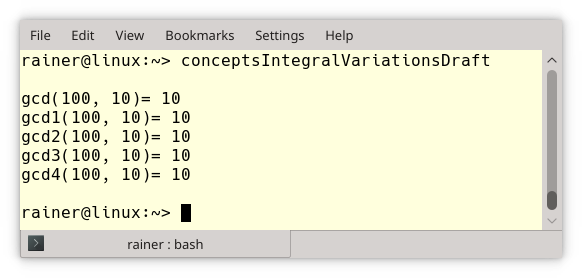An unconstrained placeholder (auto) in a function declaration generates a function template. The following two functions add are equivalent:

```template<typename T, typename T2>
return fir + sec;
}

return fir + sec;
}
```

The critical observation is that both arguments can have different types. The same holds for concepts.

```template<Arithmetic T, Arithmetic T2>                           // (1)
auto sub(T fir, T2 sec){
return fir - sec;
}

Arithmetic auto sub(Arithmetic auto fir, Arithmetic auto sec){  // (2)
return fir - sec;
}
```

The function sub requires from its arguments fir and sec,  that both support the concept Arithmetic. This means you can invoke sub(5.5, 5), and it works with the function template (line 1) and the function (line 2). The return type is deduced according to arithmetic conversion rules in both cases. The concept Arithmetic requires that fir and sec are either integral or floating-point numbers. Here is a straightforward implementation based on the type-traits function std::is_arithmetic.

```template<typename T>
concept Arithmetic = std::is_arithmetic<T>::value;
```

### A Difference between Concepts TS and Concepts Draft

I explicitly mentioned that both arguments could have different types with the Concepts Draft because this is different from the previous concepts syntax, supported by GCC. The previous syntax was based on the concepts TS (technical specification). In this syntax, the types of fir and sec have to be the same, and a call of the function sub(5.5, 5) would fail. Additionally, the previous syntax required no additional auto when concepts were used, and the definition of concepts was slightly more verbose.

```// conceptsArithmeticTS.cpp

#include <type_traits>
#include <iostream>

template<typename T>
concept bool Arithmetic(){
return std::is_arithmetic<T>::value;
}

Arithmetic sub(Arithmetic fir, Arithmetic sec){
return fir - sec;
}

int main(){

std::cout << std::endl;

std::cout << "sub(6, 5): " << sub(6, 5) << std::endl;      // (1)
std::cout << "sub(5.5, 5): " << sub(5.5, 5) << std::endl;  // (2)

std::cout << std::endl;

}
```

Because of line 2, the compilation of the program fails with the concepts TS.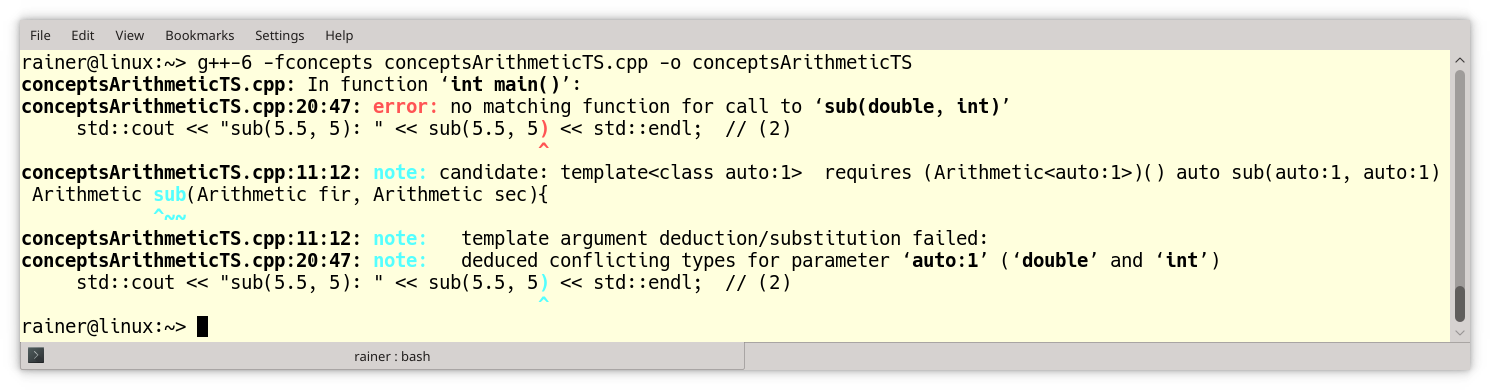The abbreviated function templates syntax behaves as expected. The new syntax support overloading. As usual, the compiler chooses the best-fitting overload.

```// conceptsOverloading.cpp

#include <type_traits>
#include <iostream>

template<typename T>
concept Integral = std::is_integral<T>::value;

std::cout << "auto : " << t << std::endl;
}

std::cout << "Integral : " << t << std::endl;
}

std::cout << "long : " << t << std::endl;
}

int main(){

std::cout << std::endl;

std::cout << std::endl;

}
```

When invoked with a double (line 1), an int (line 2), or a long int (line 3), the best-fitting overload is chosen.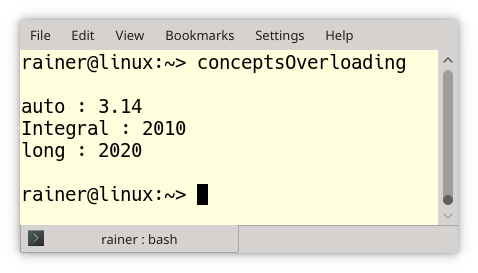## What’s next?

Two pieces are still missing in my series of concepts and are, therefore, the topic of my next posts — the definition of concepts and the so-called template introduction, which is part of the concept TS.

Thanks a lot to my Patreon Supporters: Matt Braun, Roman Postanciuc, Tobias Zindl, G Prvulovic, Reinhold Dröge, Abernitzke, Frank Grimm, Sakib, Broeserl, António Pina, Sergey Agafyin, Андрей Бурмистров, Jake, GS, Lawton Shoemake, Jozo Leko, John Breland, Venkat Nandam, Jose Francisco, Douglas Tinkham, Kuchlong Kuchlong, Robert Blanch, Truels Wissneth, Kris Kafka, Mario Luoni, Friedrich Huber, lennonli, Pramod Tikare Muralidhara, Peter Ware, Daniel Hufschläger, Alessandro Pezzato, Bob Perry, Satish Vangipuram, Andi Ireland, Richard Ohnemus, Michael Dunsky, Leo Goodstadt, John Wiederhirn, Yacob Cohen-Arazi, Florian Tischler, Robin Furness, Michael Young, Holger Detering, Bernd Mühlhaus, Matthieu Bolt, Stephen Kelley, Kyle Dean, Tusar Palauri, Dmitry Farberov, Juan Dent, George Liao, Daniel Ceperley, Jon T Hess, Stephen Totten, Wolfgang Fütterer, Matthias Grün, Phillip Diekmann, Ben Atakora, Ann Shatoff, Rob North, and Bhavith C Achar.

Thanks, in particular, to Jon Hess, Lakshman, Christian Wittenhorst, Sherhy Pyton, Dendi Suhubdy, Sudhakar Belagurusamy, Richard Sargeant, Rusty Fleming, John Nebel, Mipko, Alicja Kaminska, Slavko Radman, and David Poole.

 My special thanks to EmbarcaderoMy special thanks to PVS-StudioMy special thanks to Tipi.buildMy special thanks to Take Up Code## Seminars

I’m happy to give online seminars or face-to-face seminars worldwide. Please call me if you have any questions.

### Standard Seminars (English/German)

Here is a compilation of my standard seminars. These seminars are only meant to give you a first orientation.

• C++ – The Core Language
• C++ – The Standard Library
• C++ – Compact
• C++11 and C++14
• Concurrency with Modern C++
• Design Pattern and Architectural Pattern with C++
• Embedded Programming with Modern C++
• Generic Programming (Templates) with C++

#### New

• Clean Code with Modern C++
• C++20

### Contact Me

Modernes C++ Mentoring,Tags:
0 replies